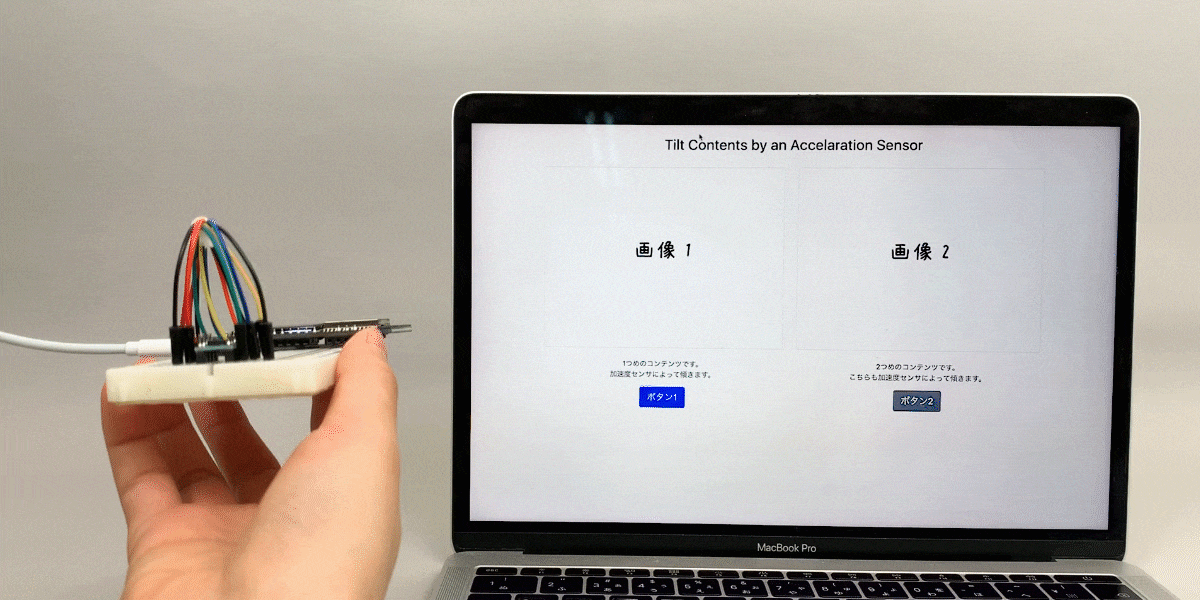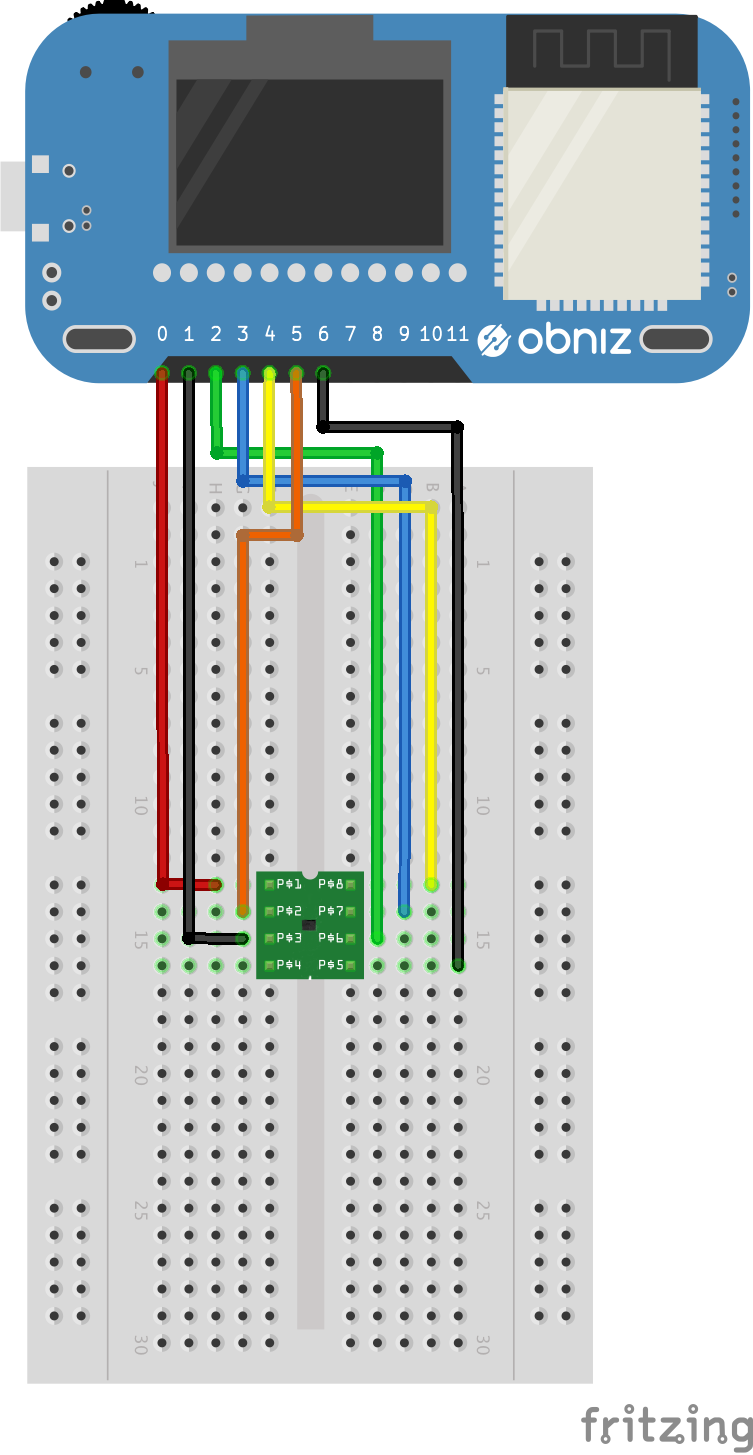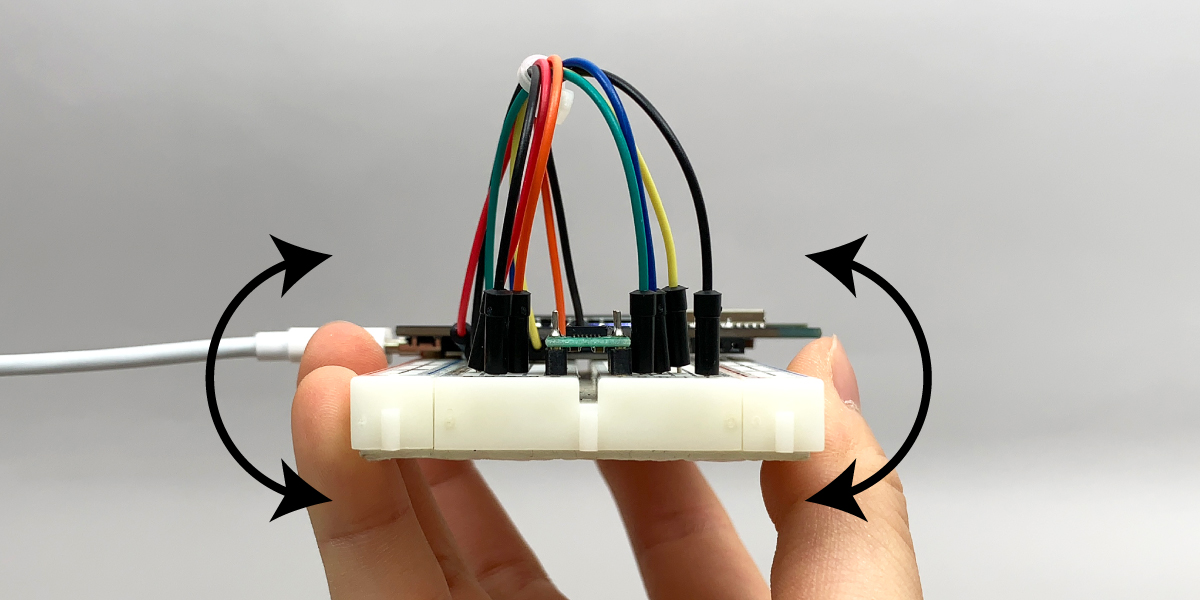## 言語切り替え# Tilt web components tilting accelerometer

コンテンツ

## Make Things

HTML elements such as images, sentences, and buttons will tilt if you tilt the acceleration sensor.

## How to make

### hardware connection

obniz Accelerometer KXR94-2050
0 Vdd(1)
1 GND(3)
2 X-axis(6)
3 Y-axis(7)
4 Z-axis(8)
5 Enable(2)
6 Self Test(5)### Software

Prepare two images, save them as “img1.jpg” and “img2.jpg“, and put them on the same folder with the code.

Input angles int the transform property of CSS to tilt HTML elements.

```\$("#img-1").css({ transform: "rotate(" + angle + "deg)" });
\$("#button-1").css({ transform: "rotate(" + angle + "deg)" });
\$("#sentence-1").css({ transform: "rotate(" + angle + "deg)" });
```

In this program, compare the first value and the current value of the acceleration sensor. Then, calculate the amount of tilt from the difference.

You can compare them with absolute values by using Math.abs()

```if (Math.abs(currentAccelVals.y - initAccelVals.y) > 0.7) {
//much tilt
} else if (Math.abs(currentAccelVals.y - initAccelVals.y) > 0.5) {
//tilt
} else if (Math.abs(currentAccelVals.y - initAccelVals.y) > 0.3) {
//little tilt
} else {
//flat
}```

## How to useWhen you rotate the Y-axis like the image above, components of the web screen tilt following your movement.